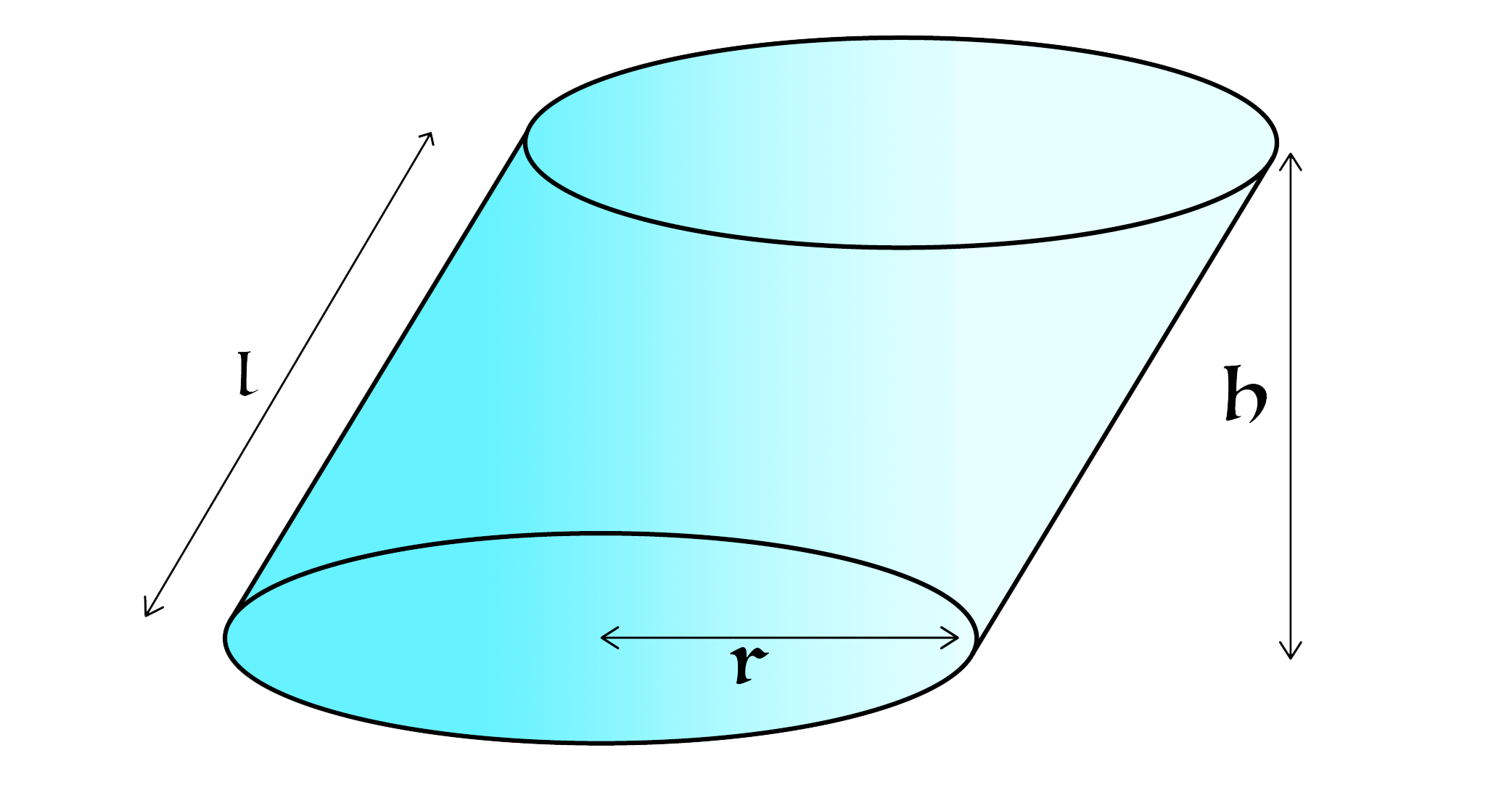# Not Your Usual Cylinder - Surface AreaWhat is the total surface area of the oblique cylinder shown above?

• The flat sides of the cylinder are circular, and are congruent and parallel to each other.
• The slant height of the cylinder is $l = 5$.
• The radius of the circular base is $r = 2$.
• The perpendicular height of the cylinder is $h = 4$.
• The image is not to scale.

State your answer to five significant figures.

Also try: Not Your Usual Cylinder - Volume

×